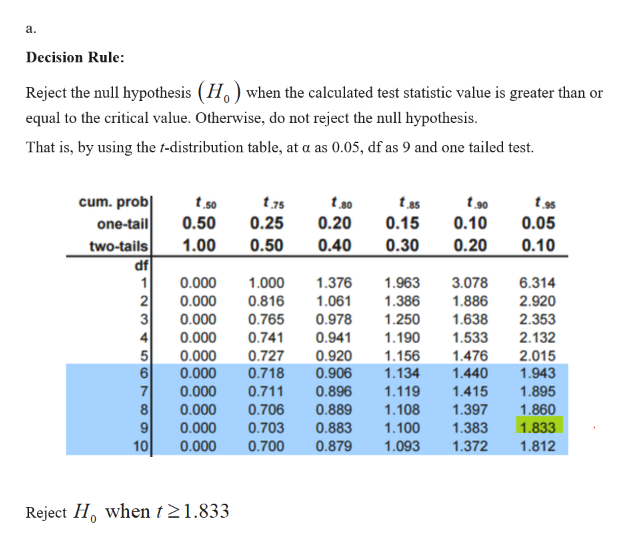# Given the following hypotheses: H0: μ ≤ 13H1: μ > 13 A random sample of 10 observations is selected from a normal population. The sample mean was 11 and the sample standard deviation 3.6. Using the 0.05 significance level:a) State the decision rule. (Round your answer to 3 decimal places.)State the decision rule________________b) Compute the value of the test statistic. (Negative answers should be indicated by a minus sign. Round your answer to 3 decimal places.)Value of the test statistic ___________________

Question
114 views

Given the following hypotheses:

H0: μ ≤ 13

H1: μ > 13

A random sample of 10 observations is selected from a normal population. The sample mean was 11 and the sample standard deviation 3.6. Using the 0.05 significance level:

a) State the decision rule. (Round your answer to 3 decimal places.)

State the decision rule________________

b) Compute the value of the test statistic. (Negative answers should be indicated by a minus sign. Round your answer to 3 decimal places.)

Value of the test statistic ___________________

check_circle

Step 1help_outlineImage Transcriptioncloseа. Decision Rule: Reject the null hypothesis (H) when the calculated test statistic value is greater than or equal to the critical value. Otherwise, do not reject the null hypothesis. That is, by using the t-distribution table, at a as 0.05, df as 9 and one tailed test. cum. prob one-tail two-tails df t75 t so 0.50 0.25 0.20 0.15 0.10 0.05 0.20 1.00 0.50 0.40 0.30 0.10 0.000 1 1.000 1.376 1.963 3.078 6.314 2 3 0.816 0.765 0.000 1.061 0.978 0.941 1.386 1.250 1.190 1.886 2.920 2.353 2.132 1.638 0.000 0.000 0.741 1.533 0.000 0.727 0.920 1.156 1.476 2.015 6 7 8 9 10 0.000 0.000 0.000 0.718 0.711 0.906 0.896 0.889 1.134 1.943 1.440 1.119 1.415 1.895 1.860 1.108 0.706 1.397 0.000 0.703 0.700 0.883 1.100 1.383 1.833 0.879 0.000 1.093 1.372 1.812 Reject H when t 21.833 LO CO N CO fullscreen

### Want to see the full answer?

See Solution

#### Want to see this answer and more?

Solutions are written by subject experts who are available 24/7. Questions are typically answered within 1 hour.*

See Solution
*Response times may vary by subject and question.
Tagged in

### Statistics